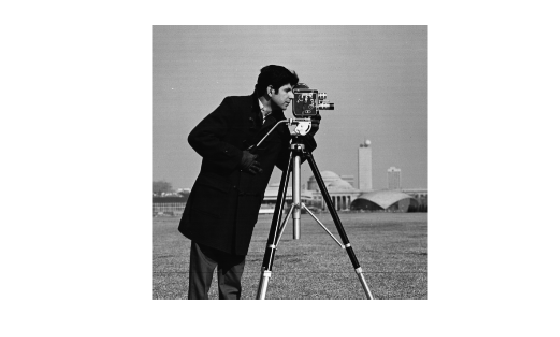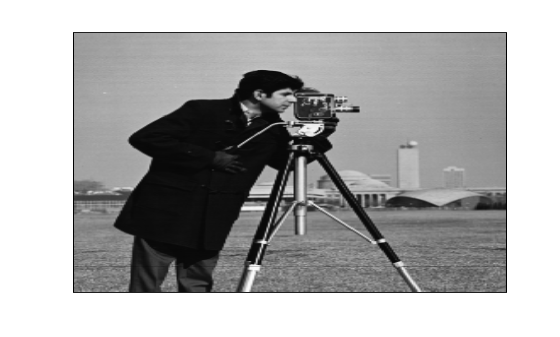# geometricTransform2d

2-D geometric transformation object

## Description

A `geometricTransform2d` object defines a custom 2-D geometric transformation using point-wise mapping functions.

## Creation

### Syntax

``tform = geometricTransform2d(inverseFcn)``
``tform = geometricTransform2d(inverseFcn,fowardFcn)``

### Description

example

````tform = geometricTransform2d(inverseFcn)` creates a `geometricTransform2d` object and sets the inverse mapping `InverseFcn` property.```
````tform = geometricTransform2d(inverseFcn,fowardFcn)` also sets the forward mapping property, `ForwardFcn`.```

## Properties

expand all

Inverse mapping function, specified as a function handle. The function should accept and return coordinates as a n-by-2 numeric matrix representing the packed (x,y) coordinates of n points.

Example: `ifcn = @(xy) [xy(:,1).^2, sqrt(xy(:,2))];`

Forward mapping function, specified as a function handle. The function should accept and return coordinates as a n-by-2 numeric matrix representing the packed (x,y) coordinates of n points.

Example: `ffcn = @(xy) [sqrt(xy(:,1)),(xy(:,2).^2)];`

## Object Functions

 `transformPointsForward` Apply forward geometric transformation `transformPointsInverse` Apply inverse geometric transformation

## Examples

collapse all

Specify the packed (x,y) coordinates of five input points. The packed coordinates are stored in a 5-by-2 matrix, where the x-coordinate of each point is in the first column, and the y-coordinate of each point is in the second column.

`XY = [10 15;11 32;15 34;2 7;2 10];`

Define the inverse mapping function. The function accepts and returns points in packed (x,y) format.

`inversefn = @(c) [c(:,1)+c(:,2),c(:,1)-c(:,2)]`
```inversefn = function_handle with value: @(c)[c(:,1)+c(:,2),c(:,1)-c(:,2)] ```

Create a 2-D geometric transform object, `tform`, that stores the inverse mapping function.

`tform = geometricTransform2d(inversefn)`
```tform = geometricTransform2d with properties: InverseFcn: @(c)[c(:,1)+c(:,2),c(:,1)-c(:,2)] ForwardFcn: [] Dimensionality: 2 ```

Apply the inverse geometric transform to the input points.

`UV = transformPointsInverse(tform,XY)`
```UV = 5×2 25 -5 43 -21 49 -19 9 -5 12 -8 ```

Specify the x- and y-coordinates vectors of five points to transform.

```x = [10 11 15 2 2]; y = [15 32 34 7 10];```

Define the inverse and forward mapping functions. Both functions accept and return points in packed (x,y) format.

```inversefn = @(c) [c(:,1).^2,sqrt(c(:,2))]; forwardfn = @(c) [sqrt(c(:,1)),c(:,2).^2];```

Create a 2-D geometric transform object, `tform`, that stores the inverse mapping function and the optional forward mapping function.

`tform = geometricTransform2d(inversefn,forwardfn)`
```tform = geometricTransform2d with properties: InverseFcn: @(c)[c(:,1).^2,sqrt(c(:,2))] ForwardFcn: @(c)[sqrt(c(:,1)),c(:,2).^2] Dimensionality: 2 ```

Apply the inverse geometric transform to the input points.

`[u,v] = transformPointsInverse(tform,x,y)`
```u = 1×5 100 121 225 4 4 ```
```v = 1×5 3.8730 5.6569 5.8310 2.6458 3.1623 ```

Apply the forward geometric transform to the transformed points `u` and `v`.

`[x,y] = transformPointsForward(tform,u,v)`
```x = 1×5 10 11 15 2 2 ```
```y = 1×5 15.0000 32.0000 34.0000 7.0000 10.0000 ```

Define an inverse mapping function that applies anisotropic scaling. The function must accept and return packed (x,y) coordinates, where the x-coordinate of each point is in the first column, and the y-coordinate of each point is in the second column.

```xscale = 0.3; yscale = 0.5; inversefn = @(xy) [xscale*xy(:,1), yscale*xy(:,2)];```

Create a 2-D geometric transform object, `tform`, that stores the inverse mapping function.

`tform = geometricTransform2d(inversefn)`
```tform = geometricTransform2d with properties: InverseFcn: @(xy)[xscale*xy(:,1),yscale*xy(:,2)] ForwardFcn: [] Dimensionality: 2 ```

Read an image to be transformed.

```I = imread('cameraman.tif'); imshow(I)```Use `imwarp` to apply the inverse geometric transform to the input image. The image is enlarged vertically by a factor of 2 (the inverse of `yscale`) and horizontally by a factor of 10/3 (the inverse of `xscale`).

```Itransformed = imwarp(I,tform); imshow(Itransformed)```Define an inverse mapping function that accepts packed (x,y) coordinates, where the x-coordinate of each point is in the first column, and the y-coordinate of each point is in the second column. The inverse mapping function in this example takes the square of the polar radial component.

```r = @(c) sqrt(c(:,1).^2 + c(:,2).^2); w = @(c) atan2(c(:,2), c(:,1)); f = @(c) [r(c).^2 .* cos(w(c)), r(c).^2 .* sin(w(c))]; g = @(c) f(c);```

Create a 2-D geometric transform object, `tform`, that stores the inverse mapping function.

`tform = geometricTransform2d(g);`

Read a color image to be transformed.

```I = imread('peppers.png'); imshow(I)```Create an `imref2d` object, specifying the size and world limits of the input and output images.

```Rin = imref2d(size(I),[-1 1],[-1 1]); Rout = imref2d(size(I),[-1 1],[-1 1]);```

Apply the inverse geometric transform to the input image.

```Itransformed = imwarp(I,Rin,tform,'OutputView',Rout); imshow(Itransformed)```